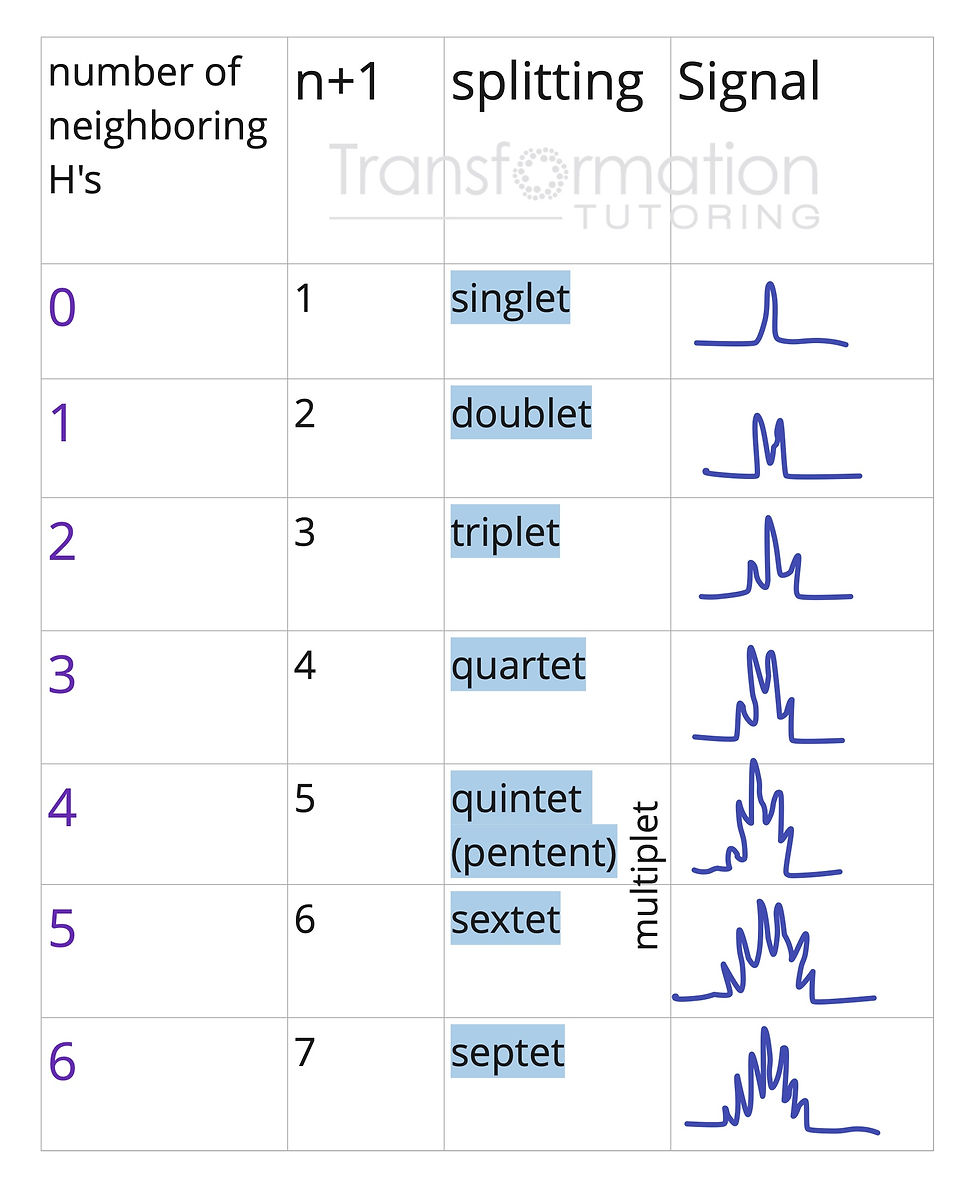top of page
Search

# Organic Chemistry by Brown, Iverson, Anslyn, Foote | Mayya's Study Guide | Chapter 13

Chapter 13: Nuclear Magnetic Resonance Spectroscopy

• Equivalent Hydrogens (Number of Signals)

• Signal Areas

• Chemical Shift

• Signal Splitting and the (n+1) Rule

• The Origins of Signal Splitting

• More Complex Splitting Patterns

• Stereochemistry and Topicity

When placed in a magnetic field, some nuclei align with it (lower energy state) and others are aligned against the magnetic field. When nuclei absorb appropriate energy, they flip from the lower energy state to the higher state in a process called resonance. The spectrometer can detect this absorption of electromagnetic radiation and records it as a signal, which is seen on the HNMR graphs we will be looking at.

When nuclei are next to electrons. These electrons create an induced magnetic field that is opposing to the external magnetic field. The proton is shielded from the external magnetic field by electrons and therefore experiences a smaller magnetic field strength. Some protons are surrounded by more electron density and are shielded while other are surrounded by less and are deshielded.

NMR spectrometer is able to measure the absorption of electromagnetic radiation as H nuclei are excited from their low energy state to the high energy state. The absorbed absorption frequencies are plotted on the HNMR graph and are shown as peaks.The 4 most important topics that we need to discuss in order to do HNMR problems correctly are:

1. Number of signals

2. Shift

3. Splitting

4. Integration

Equivalent Hydrogens (Number of Signals)

We will begin with the number of signals. Number of signals on the HNMR graph are equal to the number of different hydrogens. Hydrogens are different if they have different chemical environments (attached to different groups).Mayya's Trick:

1. Show all the hydrogens first (remember each carbon wants to have 4 bonds)

2. Look for symmetry. If you can cut a molecule in half with two identical halfs hydrogens on both sides will be identical (equivalent)

3. Hydrogens on the same carbon are usually equivalent (with some exceptions)

Signal Areas

The heights of the signals on the HNMR graph are related to the number of hydrogens they represent. The ratio of the heights of signals is equal to the ratio of the hydrogens. For example, if there are two peaks on the graph and their ratio is 3 to 1. This could mean 3H to 1H or 6H to 2H etc.

Chemical Shift

Chemical Shift allow u to determine what functional group the hydrogens are next to. For example, hydrogens on a carbon next to a carbonyl group (C=O) should be around 2-2.7ppm.

The chart is either given by the professor or has to be memorized by the students.HNMR chemical shift table (ppm values)

Signal Splitting and the (n+1) Rule

The n+1 rule predicts the splitting pattern for hydrogens on the HNMR graph, where n is the number of neighboring hydrogens.HNMR signal splitting

The Origins of Signal Splitting

When splitting occurs, the distance between the peaks in a signal is called coupling constant, J with units of Hertz. J constant values can be helpful in determining the structure of the molecule as shown below.HNMR J constant values

More Complex Splitting Patterns

What happens if the hydrogen has neighboring hydrogens that are NOT equivalent?The hydrogens in the middle have 3 neighboring H's on the left: Quartet! and 1 on the right that is not the same as the ones on the left: Doublet.

What do we get? Quartet of doublets

Vinylic hydrogens (attached to the double bond) on unsymmetrical alkenes are NOT equivalentStereochemistry and Topicity

Hydrogens on the same carbon may be : homotopic, enantiotopic or diastereotopic.

Homotopic and enantiotopic are equivalent and would not change the HNMR prediction.

Diastereotopic hydrogens are NOT equivalent and would split each other.

Steps:

1. Substitute one of the hydrogens with X. Then, substitute the other H with X

2. If the two molecules are the same, H's are homotopic. If the two molecules are enantiomers, H's are enantiotopic. If the two molecules are diastereomers, H's are diastereotopic